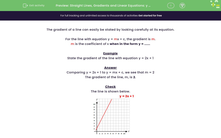# Spot the Gradient of a Straight Line from the Equation y = mx + c

In this worksheet, students will state the gradient of a straight line given its equation (y = mx + c).Key stage:  KS 3

Curriculum topic:   Algebra

Curriculum subtopic:   Use Linear Equations (Two Variables) and Gradients

Popular topics:   Algebra worksheets

Difficulty level:#### Worksheet Overview

The gradient of a line can easily be stated by looking carefully at its equation.

For the line with equation y = mx + c, the gradient is m.

m is the coefficient of x when in the form y = ......

Example

State the gradient of the line with equation y = 2x + 1

Comparing y = 2x + 1 to y = mx + c, we see that m = 2

The gradient of the line, m, is 2.

Check

The line is shown below.

y = 2x + 1Note

The equation y = 2x + 1 can be rewritten in several ways as shown below.

They all represent the same line.

y = 1 + 2x

y - 1 = 2x

x = ½ (y - 1)

Let's try some questions.

### What is EdPlace?

We're your National Curriculum aligned online education content provider helping each child succeed in English, maths and science from year 1 to GCSE. With an EdPlace account you’ll be able to track and measure progress, helping each child achieve their best. We build confidence and attainment by personalising each child’s learning at a level that suits them.

Get started